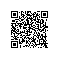# Java基础 - 原码、反码、补码

Java基础 - 原码、反码、补码

1000 0101 去除第一位符号位 1 后面的二进制转换为十进制为 5 加上第一位符号位 - 所以值为 -5

+1 = 0000 0001
-1 = 1000 0001

+1 0000 0001 0000 0001
-1 1000 0001 1111 1110

+1 0000 0001 0000 0001 0000 0001
-1 1000 0001 1111 1110 1111 1111

1 -1 = 1 + (-1) = 0000 0001(原) + 1000 0001(原) = 0000 0001(反) + 1111 1110(反) = 1111 1111(反) = 1000 0000(原) = -0

1 -1 = 1 + (-1) = 0000 0001(原) + 1000 0001(原) = 0000 0001(反) + 1111 1110(反) = 0000 0001(补) + 1111 1111(补) = (1)0000 0000(补) = 0000 0000(原) = 0

-1-127的结果应该是-128, 在用补码运算的结果中, 1000 0000(补) 就是-128. 但是注意因为实际上是使用以前的-0的补码来表示-128, 所以-128并没有原码和反码表示.对-128的补码表示1000 0000补算出来的原码是0000 0000原, 这是不正确的)

EOF使用钉钉扫一扫加入圈子
+ 订阅